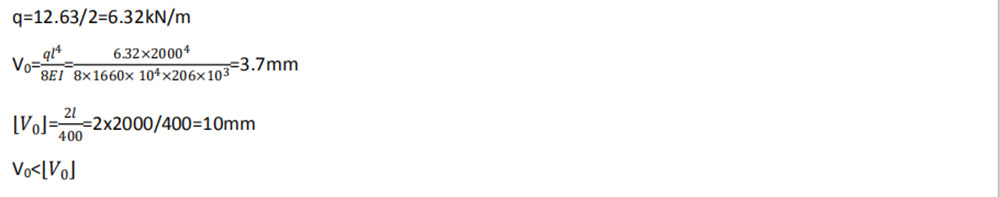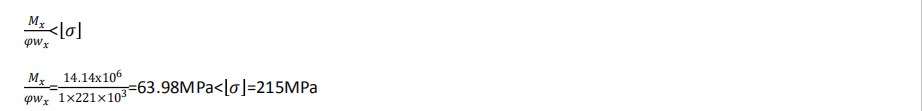# Below brief introduction for noise barrier wind load calculation for reference

Below brief introduction for noise barrier wind load calculation for reference

General situation:

For example: 2M height noise barrier; CC 2.5M distance; Install on flyover.

Fwb=Ko× K1 × K3 × Wd × Awh

Where

Fwb= Standard value of transverse wind load (kN)

W0= Reference wind pressure (kN/m2)

Wd= Design the reference wind pressure (kN/m2)

Awh=Cross windward area

V5.5= The design base wind speed in the area where the bridge is located (m/s)

Vd=Design reference wind speed at height Z (m/s)

Z= The height above the ground (m)

Y=Air gravity density (kn/m3)

K0=Design the conversion coefficient of wind speed recurrence period. For the cup beam of the large bridge with single hole span indes, k0=1.0; for other bridge k0=0.9; for Bridge in construction erection period, k0=0.75; When the bridge is located in typhoon-prone areas, the K value can be moderately increased according to the actual situation.

K3= Coefficient of terrain and geographical conditions, Generally K3=1.0.

K5=wind speed coefficient, k5=1.38 to the surface of A,B,  for the C,D which is K5=1.70.

K2=The correction coefficient of wind speed height change considering surface roughness category and gradient; the correction coefficient K2 of wind speed and height variation on the substructure of Bridges located in intermontane basins, valleys or mountain passes and other special occasions is determined according to class B ground category.

K1=wind resistance coefficient;

G= gravitational acceleration, g=9.8m/s2.

The surface roughness can be divided into A, B,C,D.

Class A refer to the offshore sea surface and the island, coast, lakeshore and desert

Class B refer to fields, villages, forests, hills, towns and suburban areas with sparse housing.

Class C refer to urban areas with dense clusters of buildings

Class D refer to an urban area with a dense cluster of buildings and higher housing.

The area belongs to Class C, the height from the ground is 8.5m, found K0=0.9; K1=1.97; K2=0.86; K5=1.70; V5.5=33m/s.

So Fwh = k0k1k3Wd Awh=12.63kN

2. Design value of horizontal force

Swh=1.4Fwh=17.68(kN)

H steel max bending monment: M= 17.68×22.5=14.14(kN·m)

H steel column is made of 150x150x7x10mm national standard steel, allowable stree =215MPa, shear stress 125MPa, Ix=1660cm4, Wx=221cm3, A=40.55cm2

3.Calculation of bending strengthMeet requirement.

4.Deflection CalculationMeet requirement

5. Whole stability calculationMeet the requirement

Above load calculation for reference.

Post time: Jul-14-2020
﻿